#Boost C++ Libraries

...one of the most highly regarded and expertly designed C++ library projects in the world.

This is the documentation for an old version of Boost. Click here to view this page for the latest version.

## TR1 C Functions Quick Reference

##### Supported TR1 Functions
```namespace boost{ namespace math{ namespace tr1{ extern "C"{

// [5.2.1.1] associated Laguerre polynomials:
double assoc_laguerre(unsigned n, unsigned m, double x);
float assoc_laguerref(unsigned n, unsigned m, float x);
long double assoc_laguerrel(unsigned n, unsigned m, long double x);

// [5.2.1.2] associated Legendre functions:
double assoc_legendre(unsigned l, unsigned m, double x);
float assoc_legendref(unsigned l, unsigned m, float x);
long double assoc_legendrel(unsigned l, unsigned m, long double x);

// [5.2.1.3] beta function:
double beta(double x, double y);
float betaf(float x, float y);
long double betal(long double x, long double y);

// [5.2.1.4] (complete) elliptic integral of the first kind:
double comp_ellint_1(double k);
float comp_ellint_1f(float k);
long double comp_ellint_1l(long double k);

// [5.2.1.5] (complete) elliptic integral of the second kind:
double comp_ellint_2(double k);
float comp_ellint_2f(float k);
long double comp_ellint_2l(long double k);

// [5.2.1.6] (complete) elliptic integral of the third kind:
double comp_ellint_3(double k, double nu);
float comp_ellint_3f(float k, float nu);
long double comp_ellint_3l(long double k, long double nu);

// [5.2.1.8] regular modified cylindrical Bessel functions:
double cyl_bessel_i(double nu, double x);
float cyl_bessel_if(float nu, float x);
long double cyl_bessel_il(long double nu, long double x);

// [5.2.1.9] cylindrical Bessel functions (of the first kind):
double cyl_bessel_j(double nu, double x);
float cyl_bessel_jf(float nu, float x);
long double cyl_bessel_jl(long double nu, long double x);

// [5.2.1.10] irregular modified cylindrical Bessel functions:
double cyl_bessel_k(double nu, double x);
float cyl_bessel_kf(float nu, float x);
long double cyl_bessel_kl(long double nu, long double x);

// [5.2.1.11] cylindrical Neumann functions;
// cylindrical Bessel functions (of the second kind):
double cyl_neumann(double nu, double x);
float cyl_neumannf(float nu, float x);
long double cyl_neumannl(long double nu, long double x);

// [5.2.1.12] (incomplete) elliptic integral of the first kind:
double ellint_1(double k, double phi);
float ellint_1f(float k, float phi);
long double ellint_1l(long double k, long double phi);

// [5.2.1.13] (incomplete) elliptic integral of the second kind:
double ellint_2(double k, double phi);
float ellint_2f(float k, float phi);
long double ellint_2l(long double k, long double phi);

// [5.2.1.14] (incomplete) elliptic integral of the third kind:
double ellint_3(double k, double nu, double phi);
float ellint_3f(float k, float nu, float phi);
long double ellint_3l(long double k, long double nu, long double phi);

// [5.2.1.15] exponential integral:
double expint(double x);
float expintf(float x);
long double expintl(long double x);

// [5.2.1.16] Hermite polynomials:
double hermite(unsigned n, double x);
float hermitef(unsigned n, float x);
long double hermitel(unsigned n, long double x);

// [5.2.1.18] Laguerre polynomials:
double laguerre(unsigned n, double x);
float laguerref(unsigned n, float x);
long double laguerrel(unsigned n, long double x);

// [5.2.1.19] Legendre polynomials:
double legendre(unsigned l, double x);
float legendref(unsigned l, float x);
long double legendrel(unsigned l, long double x);

// [5.2.1.20] Riemann zeta function:
double riemann_zeta(double);
float riemann_zetaf(float);
long double riemann_zetal(long double);

// [5.2.1.21] spherical Bessel functions (of the first kind):
double sph_bessel(unsigned n, double x);
float sph_besself(unsigned n, float x);
long double sph_bessell(unsigned n, long double x);

// [5.2.1.22] spherical associated Legendre functions:
double sph_legendre(unsigned l, unsigned m, double theta);
float sph_legendref(unsigned l, unsigned m, float theta);
long double sph_legendrel(unsigned l, unsigned m, long double theta);

// [5.2.1.23] spherical Neumann functions;
// spherical Bessel functions (of the second kind):
double sph_neumann(unsigned n, double x);
float sph_neumannf(unsigned n, float x);
long double sph_neumannl(unsigned n, long double x);

}}}} // namespaces
```

In addition sufficient additional overloads of the `double` versions of the above functions are provided, so that calling the function with any mixture of `float`, `double`, ```long double```, or integer arguments is supported, with the return type determined by the result type calculation rules.

For example:

```expintf(2.0f);  // float version, returns float.
expint(2.0f);   // also calls the float version and returns float.
expint(2.0);    // double version, returns double.
expintl(2.0L);  // long double version, returns a long double.
expint(2.0L);   // also calls the long double version.
expint(2);      // integer argument is treated as a double, returns double.
```
##### Quick Reference
```// [5.2.1.1] associated Laguerre polynomials:
double assoc_laguerre(unsigned n, unsigned m, double x);
float assoc_laguerref(unsigned n, unsigned m, float x);
long double assoc_laguerrel(unsigned n, unsigned m, long double x);
```

The assoc_laguerre functions return: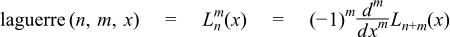See also laguerre for the full template (header only) version of this function.

```// [5.2.1.2] associated Legendre functions:
double assoc_legendre(unsigned l, unsigned m, double x);
float assoc_legendref(unsigned l, unsigned m, float x);
long double assoc_legendrel(unsigned l, unsigned m, long double x);
```

The assoc_legendre functions return: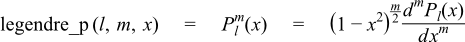See also legendre_p for the full template (header only) version of this function.

```// [5.2.1.3] beta function:
double beta(double x, double y);
float betaf(float x, float y);
long double betal(long double x, long double y);
```

Returns the beta function of x and y:See also beta for the full template (header only) version of this function.

```// [5.2.1.4] (complete) elliptic integral of the first kind:
double comp_ellint_1(double k);
float comp_ellint_1f(float k);
long double comp_ellint_1l(long double k);
```

Returns the complete elliptic integral of the first kind of k: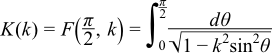See also ellint_1 for the full template (header only) version of this function.

```// [5.2.1.5] (complete) elliptic integral of the second kind:
double comp_ellint_2(double k);
float comp_ellint_2f(float k);
long double comp_ellint_2l(long double k);
```

Returns the complete elliptic integral of the second kind of k:See also ellint_2 for the full template (header only) version of this function.

```// [5.2.1.6] (complete) elliptic integral of the third kind:
double comp_ellint_3(double k, double nu);
float comp_ellint_3f(float k, float nu);
long double comp_ellint_3l(long double k, long double nu);
```

Returns the complete elliptic integral of the third kind of k and nu:See also ellint_3 for the full template (header only) version of this function.

```// [5.2.1.8] regular modified cylindrical Bessel functions:
double cyl_bessel_i(double nu, double x);
float cyl_bessel_if(float nu, float x);
long double cyl_bessel_il(long double nu, long double x);
```

Returns the modified bessel function of the first kind of nu and x:See also cyl_bessel_i for the full template (header only) version of this function.

```// [5.2.1.9] cylindrical Bessel functions (of the first kind):
double cyl_bessel_j(double nu, double x);
float cyl_bessel_jf(float nu, float x);
long double cyl_bessel_jl(long double nu, long double x);
```

Returns the bessel function of the first kind of nu and x:See also cyl_bessel_j for the full template (header only) version of this function.

```// [5.2.1.10] irregular modified cylindrical Bessel functions:
double cyl_bessel_k(double nu, double x);
float cyl_bessel_kf(float nu, float x);
long double cyl_bessel_kl(long double nu, long double x);
```

Returns the modified bessel function of the second kind of nu and x:See also cyl_bessel_k for the full template (header only) version of this function.

```// [5.2.1.11] cylindrical Neumann functions;
// cylindrical Bessel functions (of the second kind):
double cyl_neumann(double nu, double x);
float cyl_neumannf(float nu, float x);
long double cyl_neumannl(long double nu, long double x);
```

Returns the bessel function of the second kind (Neumann function) of nu and x: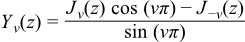See also cyl_neumann for the full template (header only) version of this function.

```// [5.2.1.12] (incomplete) elliptic integral of the first kind:
double ellint_1(double k, double phi);
float ellint_1f(float k, float phi);
long double ellint_1l(long double k, long double phi);
```

Returns the incomplete elliptic integral of the first kind of k and phi:See also ellint_1 for the full template (header only) version of this function.

```// [5.2.1.13] (incomplete) elliptic integral of the second kind:
double ellint_2(double k, double phi);
float ellint_2f(float k, float phi);
long double ellint_2l(long double k, long double phi);
```

Returns the incomplete elliptic integral of the second kind of k and phi:See also ellint_2 for the full template (header only) version of this function.

```// [5.2.1.14] (incomplete) elliptic integral of the third kind:
double ellint_3(double k, double nu, double phi);
float ellint_3f(float k, float nu, float phi);
long double ellint_3l(long double k, long double nu, long double phi);
```

Returns the incomplete elliptic integral of the third kind of k, nu and phi:See also ellint_3 for the full template (header only) version of this function.

```// [5.2.1.15] exponential integral:
double expint(double x);
float expintf(float x);
long double expintl(long double x);
```

Returns the exponential integral Ei of x:See also expint for the full template (header only) version of this function.

```// [5.2.1.16] Hermite polynomials:
double hermite(unsigned n, double x);
float hermitef(unsigned n, float x);
long double hermitel(unsigned n, long double x);
```

Returns the n'th Hermite polynomial of x:See also hermite for the full template (header only) version of this function.

```// [5.2.1.18] Laguerre polynomials:
double laguerre(unsigned n, double x);
float laguerref(unsigned n, float x);
long double laguerrel(unsigned n, long double x);
```

Returns the n'th Laguerre polynomial of x:See also laguerre for the full template (header only) version of this function.

```// [5.2.1.19] Legendre polynomials:
double legendre(unsigned l, double x);
float legendref(unsigned l, float x);
long double legendrel(unsigned l, long double x);
```

Returns the l'th Legendre polynomial of x:See also legendre_p for the full template (header only) version of this function.

```// [5.2.1.20] Riemann zeta function:
double riemann_zeta(double);
float riemann_zetaf(float);
long double riemann_zetal(long double);
```

Returns the Riemann Zeta function of x:See also zeta for the full template (header only) version of this function.

```// [5.2.1.21] spherical Bessel functions (of the first kind):
double sph_bessel(unsigned n, double x);
float sph_besself(unsigned n, float x);
long double sph_bessell(unsigned n, long double x);
```

Returns the spherical Bessel function of the first kind of x jn(x):See also sph_bessel for the full template (header only) version of this function.

```// [5.2.1.22] spherical associated Legendre functions:
double sph_legendre(unsigned l, unsigned m, double theta);
float sph_legendref(unsigned l, unsigned m, float theta);
long double sph_legendrel(unsigned l, unsigned m, long double theta);
```

Returns the spherical associated Legendre function of l, m and theta: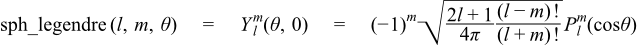See also spherical_harmonic for the full template (header only) version of this function.

```// [5.2.1.23] spherical Neumann functions;
// spherical Bessel functions (of the second kind):
double sph_neumann(unsigned n, double x);
float sph_neumannf(unsigned n, float x);
long double sph_neumannl(unsigned n, long double x);
```

Returns the spherical Neumann function of x yn(x):See also sph_bessel for the full template (header only) version of this function.

##### Currently Unsupported TR1 Functions
```// [5.2.1.7] confluent hypergeometric functions:
double conf_hyperg(double a, double c, double x);
float conf_hypergf(float a, float c, float x);
long double conf_hypergl(long double a, long double c, long double x);

// [5.2.1.17] hypergeometric functions:
double hyperg(double a, double b, double c, double x);
float hypergf(float a, float b, float c, float x);
long double hypergl(long double a, long double b, long double c,
long double x);
```
NoteThese two functions are not implemented as they are not believed to be numerically stable.
 Copyright © 2006-2010, 2012, 2013 Paul A. Bristow, Christopher Kormanyos, Hubert Holin, Bruno Lalande, John Maddock, Johan Råde, Gautam Sewani, Benjamin Sobotta, Thijs van den Berg, Daryle Walker and Xiaogang Zhang Distributed under the Boost Software License, Version 1.0. (See accompanying file LICENSE_1_0.txt or copy at http://www.boost.org/LICENSE_1_0.txt)